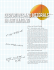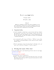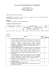# Calculus D Notes

## Transcription

Calculus D Notes
```Name: ___________________________________
www.abbymath.com - Ch. 16
Page 1 of 31
Chapter 16
Vector Calculus
16-1 & 16-5 Vector Fields, Curl, and Divergence
16-2 Line Integrals
16-3 The Fundamental Theorem for Line Integrals
16-4 Green’s Theorem
16-6 Parametric Surfaces
16-7 Surface Integrals
16-9 The Divergence Theorem
16-8 Stokes’s Theorem
16-10 Summary
The following notes are for the Calculus D (SDSU Math 252)
classes I teach at Torrey Pines High School. I wrote and
modified these notes over several semesters. The
explanations are my own; however, I borrowed several
examples and diagrams from the textbooks* my classes used
while I taught the course. Over time, I have changed some
examples and have forgotten which ones came from which
sources. Also, I have chosen to keep the notes in my own
handwriting rather than type to maintain their informality
and to avoid the tedious task of typing so many formulas,
equations, and diagrams. These notes are free for use by my
current and former students. If other calculus students and
teachers find these notes useful, I would be happy to know
that my work was helpful. - Abby Brown
SDUHSD Calculus III/D
SDSU Math 252
Abby Brown
www.abbymath.com
San Diego, CA
*Calculus: Early Transcendentals, 6th & 4th editions, James Stewart, ©2007 & 1999
Brooks/Cole Publishing Company, ISBN 0-495-01166-5 & 0-534-36298-2.
(Chapter, section, page, and formula numbers refer to the 6th edition of this text.)
*Calculus, 5th edition, Roland E. Larson, Robert P. Hostetler, & Bruce H. Edwards, ©1994
D. C. Heath and Company, ISBN 0-669-35335-3.
Name: ___________________________________
www.abbymath.com - Ch. 16
Page 2 of 31
Name: ___________________________________
www.abbymath.com - Ch. 16
Page 3 of 31
Name: ___________________________________
www.abbymath.com - Ch. 16
Page 4 of 31
Name: ___________________________________
www.abbymath.com - Ch. 16
Page 5 of 31
Name: ___________________________________
www.abbymath.com - Ch. 16
Page 6 of 31
Name: ___________________________________
www.abbymath.com - Ch. 16
Page 7 of 31
Name: ___________________________________
www.abbymath.com - Ch. 16
Page 8 of 31
Name: ___________________________________
www.abbymath.com - Ch. 16
Page 9 of 31
Name: ___________________________________
www.abbymath.com - Ch. 16
Page 10 of 31
Name: ___________________________________
www.abbymath.com - Ch. 16
Page 11 of 31
Name: ___________________________________
www.abbymath.com - Ch. 16
Page 12 of 31
Name: ___________________________________
www.abbymath.com - Ch. 16
Page 13 of 31
Name: ___________________________________
www.abbymath.com - Ch. 16
Page 14 of 31
Name: ___________________________________
www.abbymath.com - Ch. 16
Page 15 of 31
Name: ___________________________________
www.abbymath.com - Ch. 16
Page 16 of 31
Name: ___________________________________
www.abbymath.com - Ch. 16
Page 17 of 31
Name: ___________________________________
www.abbymath.com - Ch. 16
Page 18 of 31
Name: ___________________________________
www.abbymath.com - Ch. 16
Page 19 of 31
Name: ___________________________________
www.abbymath.com - Ch. 16
Page 20 of 31
Name: ___________________________________
www.abbymath.com - Ch. 16
Page 21 of 31
Name: ___________________________________
www.abbymath.com - Ch. 16
Page 22 of 31
Name: ___________________________________
www.abbymath.com - Ch. 16
Page 23 of 31
Name: ___________________________________
www.abbymath.com - Ch. 16
Page 24 of 31
Name: ___________________________________
www.abbymath.com - Ch. 16
Page 25 of 31
Name: ___________________________________
www.abbymath.com - Ch. 16
Page 26 of 31
Name: ___________________________________
www.abbymath.com - Ch. 16
Page 27 of 31
Name: ___________________________________
www.abbymath.com - Ch. 16
Page 28 of 31
Name: ___________________________________
www.abbymath.com - Ch. 16
Page 29 of 31
Name: ___________________________________
www.abbymath.com - Ch. 16
Page 30 of 31
Name: ___________________________________
www.abbymath.com - Ch. 16
Page 31 of 31
Integration Summary
Scalar Functions
z
zz
b
interval length =
A=
dx
a
A=
V =
dA
R
V = ∫∫∫ dV
z
zz
b
a
f dx
f dA
z
= ds
arc length = s
=
surface area = S
R
mass
zz
“curtain” area or mass =
C
dS
mass of surface lamina =
S
= ∫∫∫ f dV
z
zz
C
f ds
f dS
S
Note: Integral represents “mass” if f is a density function.
E
E
Vector Functions
work = ∫ F ⋅ dr
flux
C
zz
zz
zz
zz
=
F ⋅ dS
S
= ∫ F ⋅ T ds
=
C
= ∫ F ⋅ r′(t ) dt
=
a
= ∫ P dx + Q dy + R dz ← differential form
r ′(t )
r ′(t )
N=
∇G
∇G
F ⋅ N dS
S
b
T=
F ⋅∇G dA
R
C
=
F ⋅ (ru × rv ) dA ← parametric form
D
Elements of Integration
dA = dy dx, r dr d2, du dv
dV = dz dy dx, r dz dr d2, D2sinN dD dN d2
dr = T ds = r ′ ( t ) dt
dS = N dS = ∇G dA
ds = r ′( t ) dt = [ x ′ ( t )]2 + [ y ′ ( t )]2 + [ z ′ ( t )]2 dt
dS = ∇G dA = [ g x ( x , y )]2 + [ g y ( x , y )]2 + 1 dA where z = g ( x , y ) and G ( x , y , z ) = z − g ( x , y )
= ru × rv dA where S is given by r ( u, v ) ← parametric form
F
C
Closed
z
C
Conservative
(› a potential function f such that F=Lf)
F ⋅ dr = 0
Note: Green’s, Stokes’s, and
Fundamental Theorem also
apply in this case.
Not Conservative
Green’s Theorem (2D)
∫
Fundamental Theorem of Line Integrals
Not
Closed
z
F ⋅ dr = f ( x ( b), y ( b), z ( b))
− f ( x ( a ), y ( a ), z ( a ))
C
where F = ∇ f
If S is closed: Divergence Theorem
∫∫ F ⋅ dS = ∫∫∫ div F dV
S
E
C
z
C
F ⋅ dr = ∫∫
R
∂Q ∂P
−
dA
∂x ∂y
z
Stokes’s Theorem (3D)
∫
C
F ⋅ dr = ∫∫ curl F ⋅ dS
S
b
F ⋅ dr = F ⋅ r ′( t ) dt
a
Complete the line integral
www.abbymath.com
Abby Brown ~ 11/2003
```

### Calculus D Notes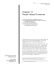and to avoid the tedious task of typing so many formulas, equations, and diagrams. These notes are free for use by my current and former students. If other calculus students and teachers find these...

### Calculus D Notes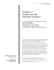and to avoid the tedious task of typing so many formulas, equations, and diagrams. These notes are free for use by my current and former students. If other calculus students and teachers find these...

### Calculus D Notes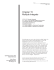and to avoid the tedious task of typing so many formulas, equations, and diagrams. These notes are free for use by my current and former students. If other calculus students and teachers find these...

### Worksheet 23: Stokes and Divergence Theorems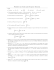### Calculus C Notes - Chapters 7 and 8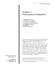and to avoid the tedious task of typing so many formulas, equations, and diagrams. These notes are free for use by my current and former students. If other calculus students and teachers find these...

### Math 3Z03 Assignment #3 Due: Monday, February 23rd, 2015 Solve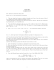### 6 Theme The Integral as an Accumulation Function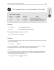### 1.6 Exam advice and sample questions for Chapter 1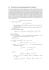### Homework 8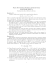### Session 7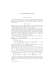### Sample Final Exam #1 Math 3C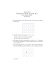### Syllabus - Polytechnic University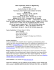### Mathematical Modeling as a Means to Demonstrate the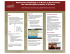### Math 201 Course Guidelines - Department of Mathematics, CCNY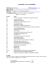### What mathematics is hidden behind the### Math205 MM syllabus - Department of Mathematics, CCNY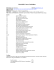### On strongly monotone flows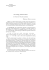### WELCOME TO 1E1! Calculus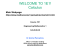### Summer Work for Students Entering AP Calculus AB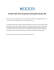### Derivatives and integrals in art imaging